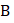# Two men ‘’ and ‘’ are standing on a plank. ‘’ is at the middle of the plank andis at the left end of the plank. Lower surface of the plank is smooth. System is initially at rest and masses are as shown in fig. ‘’  and ‘’ start moving such that the position ofremains fixed with respect to ground, then ‘’ meetsThen the point wheremeetsis located ata) The middle of the plank b) 30 cm from the left end of the plank c) The right end of the plank d) None of these

## Question ID - 100227 :- Two men ‘’ and ‘’ are standing on a plank. ‘’ is at the middle of the plank andis at the left end of the plank. Lower surface of the plank is smooth. System is initially at rest and masses are as shown in fig. ‘’  and ‘’ start moving such that the position ofremains fixed with respect to ground, then ‘’ meetsThen the point wheremeetsis located ata) The middle of the plank b) 30 cm from the left end of the plank c) The right end of the plank d) None of these

3537

(c)

Taking the origin at the center of the plank,(Assuming the centres of the two men are exactly at the axis as shown in fig.)Hence,andmeet at the right end of the plank

Next Question :
 Two particles of equal masses have velocitiesm/s andat any instant. The first particle has a constant accelerationwhile the acceleration of the other particle is zero. The centre of mass of the two particles moves in a a) Circle b) Parabola c) Straight line d) Ellipse